Wacker Art Fractal Generator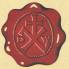German Version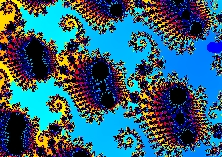Mandelbrot Fractal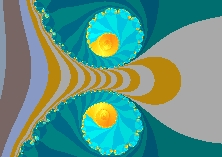Julia FractalMandelbrot FractalJulia FractalJulia FractalMandelbrot Fractal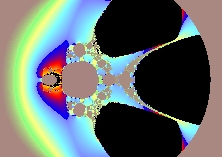Fractal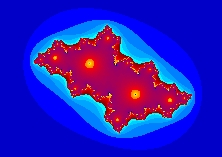Julia FractalJulia Fractal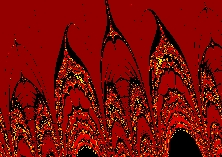Julia FractalJulia FractalZ-2 - Z1FractalJulia FractalJulia Fractal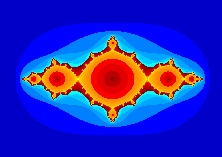Julia FractalJulia FractalBurning Ship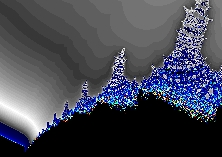Chines TowerMandelbrot FractalMandelbrot Fraktal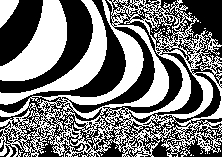Mandelbrot Fraktal

 Tipps: How to use the Wacker Art Fractal GeneratorMove the Courser to the part of the fractal you are interested in, and click with the left mouse button at the centre part of this area. The fractal will be recalculated in a zoom in version with a 1:2 zoom ratio, with the click point as new centre point. Use the right mouse button for zoom out.

Press the"Zoom Out" Button to get a view of the surrounding area of the displayed fractal.

If the black part of the fractal is too rough, it is proposed to raise the number of iterations. To do this press the "+" button. This will double the number of iterations and start the recalculation of the fractal with the new parameter.

However, be aware that the the calculation time of the fractal increases with the numberof iterations. So use care when pressing the "+" button.

When the "-" button is pressed the calculation will be restarted with half the number of iterations.

If you like to have a printed version of the fractal, press the "Print" button. This will lead to a recalculation of the fractal to the selected page format and is time consuming.

With the "Full Screen Window" Button it is possible to get the fractal displayed in a separate Java-Window, with the maximum possible size of the actual screen.

Julia Fractals

Each fractal generated by the fractal generator is a map of julia fractals.
For each point of a fractal exists an assigned julia fractal.
With the fractal generator it is possible to calculate these fractals. There are two ways for generating julia fractals:

1.) Select the item Julia Selector in the algorithem menu. The curser will change to the crosshair curser +.
If you click with this curser on a point of the fractal image, the assigned julia fractal will be calculated.
In the algorithem menu the item Julia Selector has changed to Fractal Map when the calculation of the julia fractal has finished. With this menu item it is possible to go back to the original fractal.

2.) Each fractal is part of the plane of complex numbers. Instead of selecting the complex number with the curser it is possible to enter the complex value of a julia fractal by the Set Julia Value window. Go to the algorithem menu and select the Set Julia Value item for the display of the window and enter the complex value of a julia fractal.

Java Plugins

Information on Java Plugins can be found on the Wacker Art RGB Color Mixer Page.

Fractal Gallery and JavaScript

To get the fractals of the fractal gallery calculated in a separate Java window, JavaScript has to be activated in your browser.

 Wacker Art Fractal GalleryVisit the interactive Wacker Art Fractal Gallery. You can do a mouse click on one of the fractal icons and the fractal will be calculated in a seperate Java window. In this window the fractal can be further explored. You can zoom-in and zoom-out or choose a different layout.

The icons in the right coloum of this page use the same algorithm. You can test this algorithm of the fractal generator by clicking on one of the icons.

 Wacker Art Fractal CatalogAn overview on fractals created by different algorithms can be found through the following links.

Click on one of the following icons to come to a page of the Wacker Art Fractal Catalog.

 Fractal Algorithm DefinitionUse the following links to come to the algorithm definition pages. On this pages it is possible to define different algorithms for the calculation of fractals.

Click on one of the icons to come to a algoritm definition page.

 Burning ShipCalculate fascinating fractals using the "Burning Ship" algorithm

Click on the picture below to come to my "Burning Ship" internet page.

 Celtic MandelbrotCalculate fascinating fractals using the "Celtic Mandelbrot" algorithm

Click on the picture below to come to my "Celtic Mandelbrot" internet page.

30. June 2010 Version 2.1
Copyright: Hermann Wacker Uhlandstraße 10 D-85386 Eching bei Freising Germany Disclaimer